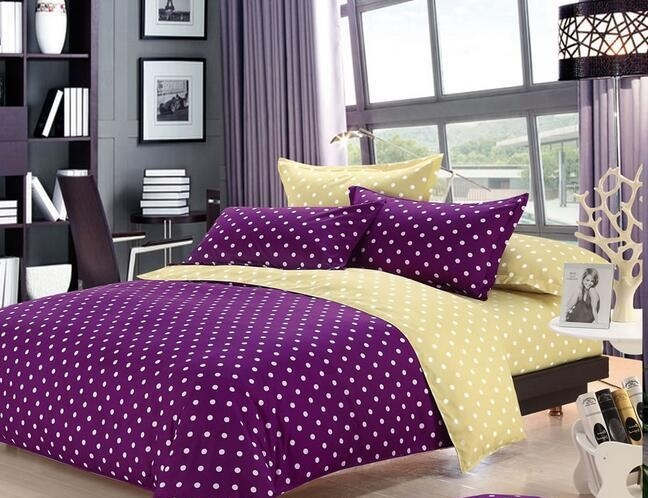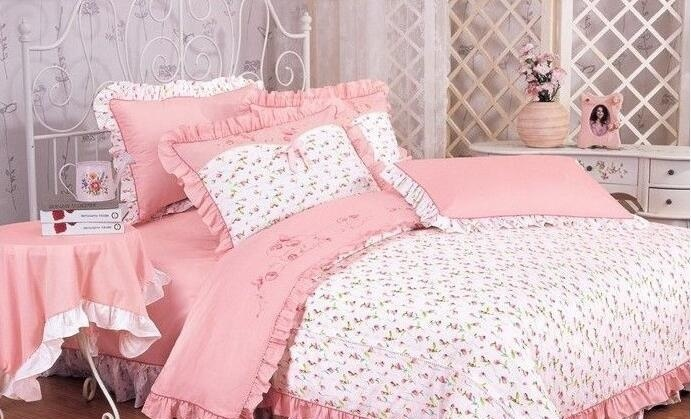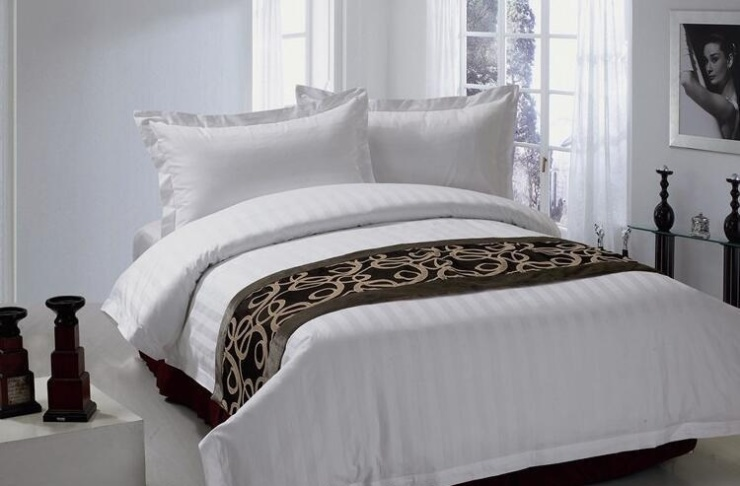|

# 南方寝室床品怎么样 价格贵不贵

又是一年冬季，现在很多家庭都在忙着为自己和家庭人添床被子，在寒冷的冬季被窝是每个人的珍宝，好的寝室床品可以带给我们舒适健康体验，为私密空间增彩添色，今天为大家介绍国内知名的南方寝室床品怎么样？价格贵不贵？南方寝室床品品牌介绍

南方寝饰科技有限公司，创始于1981年，针对25－45都市女性需求研制销售家纺产品的综合性企业，享有“喜庆床品专家”之美誉。

南方寝饰创始于1981年，是国内知名家纺企业，拥有中国家纺行业“喜庆床品专家”美誉。2010年，南方寝饰携手意大利路易家族推出全新子品牌“MACARY”曼卡蕾，专做国内礼品市场。

南方寝饰以品牌为旗帜，传统文化为依托，更与现代时尚韵律相依托，坚持华贵优质的品牌路线，以喜庆床品专家为诉求点，发展成为25－45岁都市女性为主大众消费的综合性城市家纺品牌，拥有喜庆系列、居家系列、青少年系列、芯类系列及其他系列等5大品类，涵盖了床罩多件套类、床单四件套类、被子、枕芯、毛浴巾类、靠垫类、夏令产品类、儿童用品类、床垫类、单件组合类等10大系列300多个品种的产品，满足广大消费者居家购物选择。

2002年，第一家＂南方寝饰＂专卖店开设于浙江省温州市，目前，南方寝饰连锁销售网络遍布全国30个省市自治区，在全国开设加盟专卖店、专柜1000多家，为越来越多的经销商所选择、为越来越多的消费者所认可。

公司从创立之日起，始终坚持实行严格的品牌战略管理体制，先后通过了ISO9001：2008质量管理认证，取得生态纤维制品标志使用准用证，2007年荣膺＂浙江名牌产品＂等荣誉称号。2008年荣获2007－2008温州针棉织品领军品牌，获中国色彩流行协会＂中国家用纺织品色彩应用示范基地＂称号。2009年被评为＂中国最具流行魅力家用纺织品品牌＂，2010年被授予＂中国纺织服装商业杰出企业＂、＂中国礼品行业TOP 30杰出品牌＂荣誉称号，并再度获批成为＂中国喜庆床品色彩研发基地＂。南方寝饰床品价格

南方寝饰床品分为各类套件、被子、毛毯、枕芯等产品，各种南方寑饰床品价格参考如下。

南方寑饰床品套件主要分为豪华的和普通两大类。上等的床品套件价格在1000元以上，主要是一些比较好的婚庆床品和好材质好手工的床品。普通套件类价格相对较便宜，两三百元是比较普遍的，便宜一点的就一两百元了。南方寑饰便宜的被子价格在一两百左右，稍微好一些的价格在三四百左右，里面的填充材质是鸭绒、羊绒棉之类的上等材质的价格会上到八百甚至千元。南方寑饰比较常见的普通枕芯价格都在百元以下，保健枕和一些羽绒枕的价格会高一些，上到百元。

床上用品购买攻略

一、床上用品简介

床上用品，包括“芯”和“套”。 很多新人弄不清楚“床上N件套”到底是什么，在详细介绍之前，小编先来说说床上用品具体是什么。通常说的“床上几件套”，指的是被套、枕套、床单。四件套就是1个被套，2个枕套，1个床单。有的六件套、八件套，会有靠垫的布套。 床上几件套，并不是被子、枕头，被芯和枕芯这些是要单买的。

二、被芯

以前的被芯都是棉花，现在的被芯，种类繁多。最常见的就是太空棉、纤维、蚕丝、这几种被芯都比棉花要轻很多，而且又很好的保暖效果。羊毛、羽绒、羊绒、驼毛、驼绒、羽毛等材质也被做成被芯，供新人选择。 被芯最好选择天然材质，像是近年来流行的蚕丝被，一年四季都可以用。

1、蚕丝被

小编首先推荐蚕丝被。透气、轻软、舒适是蚕丝被最大的特点，有人甚至形容它是人的第二层肌肤。蚕丝具有非常高的吸湿、透气性，被人们称为“纤维皇后”。蚕丝被可以让人体肌肤自由地排汗、分泌，保持皮肤清洁。 蚕丝被轻薄，冬天也只需要选择单人被2公斤左右，双人被2.5公斤左右的即可完全保暖了。 对新人而言，选择蚕丝被，亲密接触无障碍。

2、羽绒被

对生活品质要求很高的新人，不妨选择好的羽绒被。羽绒被有很好的保暖性，盖起来不会有闷热感，还能吸湿排汗。同样体积下的羽绒被，分量只有1－1.5公斤，是非常轻的。 羽绒被非常耐用，不变形，保养得当的话，能用30年左右。对羽绒类过敏的新人，请慎重选择。

3、羊毛被

羊毛自身带有垂悬的特性，因此非常贴身舒适。由于卷曲的羊毛中，含有大量的空气；而空气的传热率非常低，能有效的防止外部冷空气的进入与内部热空气的散发，因而能达到很高的保温性。富有弹性不易沾污，清洁起来很方便。但羊毛被的透气性要比羽绒被和蚕丝被差一些，也更重一些。 新人们可以将羊毛被当做被褥来使用，睡在上面是非常舒服的。

4、驼毛被

用驼毛做成的被子除了保暖外，还具有立体感，很显档次。上等驼毛被选用纯驼毛经过高温特殊处理后制作而成，驼毛纤维细度高，保暖性极佳，透气性好，有良好的弹性与蓬松感，适于在阴湿寒冷地区使用。 像上海冬季阴冷潮湿，房间内也没有暖气，新人在准备冬季被子的时候，可以优先考虑驼毛被。三、被套

被套从几十块到几千块都有，为什么有这么大的价格差呢？被套的材质不同，价格自然不同。买被套要注意以下几点：

1、面料。被套贴身盖，要选择百分百纯棉。最好选择斜纹纯棉的布料，手感密实厚重。小编教大家一个不能让商家看到的办法，就是偷偷用手指沾一点水轻轻擦拭被套，看看会不会掉色。

2、尺寸。是否足寸。有些被套会比实际标明的小一点。在量的时候，如果尺寸比标注的稍微大了一些，这是商家考虑周到，因为纯棉制品会有缩水的现象。

3、这里还有一个关于被套的小秘密。翻开被罩的四个角，看看有没有系被子的小绳子。质量好的产品，会在这些细节上做足功夫。

四、枕芯

枕头作为床上用品，也越来越被重视，而且价格不菲的枕芯比比皆是。像是新推出的记忆枕，不但枕起来很舒适，而且对肩部也能起到放松的作用。市面上常见的枕芯有：九孔枕、纤维枕、蚕丝枕、记忆枕、羽绒枕、蚕砂枕等等。枕头质地要柔软，透气性好，不宜过高过低，以8～15厘米高为宜。小编建议先去实体店试验一下，看哪种枕头舒服，再去网上购买。枕着不舒服的枕头睡觉，是非常郁闷的。

小编提示：并不是价格越高，“高科技”含量越高的枕芯就是好的，很多都是商家招揽生意的噱头。

五、枕套

枕套的面料以轻柔为好，所以多选用纯棉制品。枕套的手感要好，你在侧卧时脸部会贴在枕头上，面料不舒服人会很难受。细节上，拉链的地方要有多余出来的布料挡住，这样才不会挂到床单。单独挑选枕套时，最好能带着枕头一起去，当面试一试，防止买回家套不进去，尺寸有时候也会出现偏差的。

六、尺寸和颜色

讲完了细节，小编来说说床上用品的尺寸和颜色。尺寸要根据已经买好的婚床和生活习惯来定，有的夫妻习惯一人一个单人被，有的则是双人被。布置新人的房间，当然要以红色为主，但是大红色其实并不是很实用。新人可以买一套便宜点的大红色四件套，结婚当天用用就算了。日常用起来，还是淡雅耐脏的颜色为好。

`声明：本文由入驻焦点开放平台的作者撰写，除焦点官方账号外，观点仅代表作者本人，不代表焦点立场错误信息举报电话： 400-099-0099，邮箱：jubao@vip.sohu.com，或点此进行意见反馈，或点此进行举报投诉。`A B C D E F G H J K L M N P Q R S T W X Y Z
A - B - C - D - E
• A
• 鞍山
• 安庆
• 安阳
• 安顺
• 安康
• 澳门
• B
• 北京
• 保定
• 包头
• 巴彦淖尔
• 本溪
• 蚌埠
• 亳州
• 滨州
• 北海
• 百色
• 巴中
• 毕节
• 保山
• 宝鸡
• 白银
• 巴州
• C
• 承德
• 沧州
• 长治
• 赤峰
• 朝阳
• 长春
• 常州
• 滁州
• 池州
• 长沙
• 常德
• 郴州
• 潮州
• 崇左
• 重庆
• 成都
• 楚雄
• 昌都
• 慈溪
• 常熟
• D
• 大同
• 大连
• 丹东
• 大庆
• 东营
• 德州
• 东莞
• 德阳
• 达州
• 大理
• 德宏
• 定西
• 儋州
• 东平
• E
• 鄂尔多斯
• 鄂州
• 恩施
F - G - H - I - J
• F
• 抚顺
• 阜新
• 阜阳
• 福州
• 抚州
• 佛山
• 防城港
• G
• 赣州
• 广州
• 桂林
• 贵港
• 广元
• 广安
• 贵阳
• 固原
• H
• 邯郸
• 衡水
• 呼和浩特
• 呼伦贝尔
• 葫芦岛
• 哈尔滨
• 黑河
• 淮安
• 杭州
• 湖州
• 合肥
• 淮南
• 淮北
• 黄山
• 菏泽
• 鹤壁
• 黄石
• 黄冈
• 衡阳
• 怀化
• 惠州
• 河源
• 贺州
• 河池
• 海口
• 红河
• 汉中
• 海东
• I
• J
• 晋中
• 锦州
• 吉林
• 鸡西
• 佳木斯
• 嘉兴
• 金华
• 景德镇
• 九江
• 吉安
• 济南
• 济宁
• 焦作
• 荆门
• 荆州
• 江门
• 揭阳
• 金昌
• 酒泉
• 嘉峪关
K - L - M - N - P
• K
• 开封
• 昆明
• 昆山
• L
• 廊坊
• 临汾
• 辽阳
• 连云港
• 丽水
• 六安
• 龙岩
• 莱芜
• 临沂
• 聊城
• 洛阳
• 漯河
• 娄底
• 柳州
• 来宾
• 泸州
• 乐山
• 六盘水
• 丽江
• 临沧
• 拉萨
• 林芝
• 兰州
• 陇南
• M
• 牡丹江
• 马鞍山
• 茂名
• 梅州
• 绵阳
• 眉山
• N
• 南京
• 南通
• 宁波
• 南平
• 宁德
• 南昌
• 南阳
• 南宁
• 内江
• 南充
• P
• 盘锦
• 莆田
• 平顶山
• 濮阳
• 攀枝花
• 普洱
• 平凉
Q - R - S - T - W
• Q
• 秦皇岛
• 齐齐哈尔
• 衢州
• 泉州
• 青岛
• 清远
• 钦州
• 黔南
• 曲靖
• 庆阳
• R
• 日照
• 日喀则
• S
• 石家庄
• 沈阳
• 双鸭山
• 绥化
• 上海
• 苏州
• 宿迁
• 绍兴
• 宿州
• 三明
• 上饶
• 三门峡
• 商丘
• 十堰
• 随州
• 邵阳
• 韶关
• 深圳
• 汕头
• 汕尾
• 三亚
• 三沙
• 遂宁
• 山南
• 商洛
• 石嘴山
• T
• 天津
• 唐山
• 太原
• 通辽
• 铁岭
• 泰州
• 台州
• 铜陵
• 泰安
• 铜仁
• 铜川
• 天水
• 天门
• W
• 乌海
• 乌兰察布
• 无锡
• 温州
• 芜湖
• 潍坊
• 威海
• 武汉
• 梧州
• 渭南
• 武威
• 吴忠
• 乌鲁木齐
X - Y - Z
• X
• 邢台
• 徐州
• 宣城
• 厦门
• 新乡
• 许昌
• 信阳
• 襄阳
• 孝感
• 咸宁
• 湘潭
• 湘西
• 西双版纳
• 西安
• 咸阳
• 西宁
• 仙桃
• 西昌
• Y
• 运城
• 营口
• 盐城
• 扬州
• 鹰潭
• 宜春
• 烟台
• 宜昌
• 岳阳
• 益阳
• 永州
• 阳江
• 云浮
• 玉林
• 宜宾
• 雅安
• 玉溪
• 延安
• 榆林
• 银川
• Z
• 张家口
• 镇江
• 舟山
• 漳州
• 淄博
• 枣庄
• 郑州
• 周口
• 驻马店
• 株洲
• 张家界
• 珠海
• 湛江
• 肇庆
• 中山
• 自贡
• 资阳
• 遵义
• 昭通
• 张掖
• 中卫

1室1厅1厨1卫1阳台

1
2
3
4
5

0
1
2

1

1

0
1
2
3报名成功，资料已提交审核A B C D E F G H J K L M N P Q R S T W X Y Z
A - B - C - D - E
• A
• 鞍山
• 安庆
• 安阳
• 安顺
• 安康
• 澳门
• B
• 北京
• 保定
• 包头
• 巴彦淖尔
• 本溪
• 蚌埠
• 亳州
• 滨州
• 北海
• 百色
• 巴中
• 毕节
• 保山
• 宝鸡
• 白银
• 巴州
• C
• 承德
• 沧州
• 长治
• 赤峰
• 朝阳
• 长春
• 常州
• 滁州
• 池州
• 长沙
• 常德
• 郴州
• 潮州
• 崇左
• 重庆
• 成都
• 楚雄
• 昌都
• 慈溪
• 常熟
• D
• 大同
• 大连
• 丹东
• 大庆
• 东营
• 德州
• 东莞
• 德阳
• 达州
• 大理
• 德宏
• 定西
• 儋州
• 东平
• E
• 鄂尔多斯
• 鄂州
• 恩施
F - G - H - I - J
• F
• 抚顺
• 阜新
• 阜阳
• 福州
• 抚州
• 佛山
• 防城港
• G
• 赣州
• 广州
• 桂林
• 贵港
• 广元
• 广安
• 贵阳
• 固原
• H
• 邯郸
• 衡水
• 呼和浩特
• 呼伦贝尔
• 葫芦岛
• 哈尔滨
• 黑河
• 淮安
• 杭州
• 湖州
• 合肥
• 淮南
• 淮北
• 黄山
• 菏泽
• 鹤壁
• 黄石
• 黄冈
• 衡阳
• 怀化
• 惠州
• 河源
• 贺州
• 河池
• 海口
• 红河
• 汉中
• 海东
• I
• J
• 晋中
• 锦州
• 吉林
• 鸡西
• 佳木斯
• 嘉兴
• 金华
• 景德镇
• 九江
• 吉安
• 济南
• 济宁
• 焦作
• 荆门
• 荆州
• 江门
• 揭阳
• 金昌
• 酒泉
• 嘉峪关
K - L - M - N - P
• K
• 开封
• 昆明
• 昆山
• L
• 廊坊
• 临汾
• 辽阳
• 连云港
• 丽水
• 六安
• 龙岩
• 莱芜
• 临沂
• 聊城
• 洛阳
• 漯河
• 娄底
• 柳州
• 来宾
• 泸州
• 乐山
• 六盘水
• 丽江
• 临沧
• 拉萨
• 林芝
• 兰州
• 陇南
• M
• 牡丹江
• 马鞍山
• 茂名
• 梅州
• 绵阳
• 眉山
• N
• 南京
• 南通
• 宁波
• 南平
• 宁德
• 南昌
• 南阳
• 南宁
• 内江
• 南充
• P
• 盘锦
• 莆田
• 平顶山
• 濮阳
• 攀枝花
• 普洱
• 平凉
Q - R - S - T - W
• Q
• 秦皇岛
• 齐齐哈尔
• 衢州
• 泉州
• 青岛
• 清远
• 钦州
• 黔南
• 曲靖
• 庆阳
• R
• 日照
• 日喀则
• S
• 石家庄
• 沈阳
• 双鸭山
• 绥化
• 上海
• 苏州
• 宿迁
• 绍兴
• 宿州
• 三明
• 上饶
• 三门峡
• 商丘
• 十堰
• 随州
• 邵阳
• 韶关
• 深圳
• 汕头
• 汕尾
• 三亚
• 三沙
• 遂宁
• 山南
• 商洛
• 石嘴山
• T
• 天津
• 唐山
• 太原
• 通辽
• 铁岭
• 泰州
• 台州
• 铜陵
• 泰安
• 铜仁
• 铜川
• 天水
• 天门
• W
• 乌海
• 乌兰察布
• 无锡
• 温州
• 芜湖
• 潍坊
• 威海
• 武汉
• 梧州
• 渭南
• 武威
• 吴忠
• 乌鲁木齐
X - Y - Z
• X
• 邢台
• 徐州
• 宣城
• 厦门
• 新乡
• 许昌
• 信阳
• 襄阳
• 孝感
• 咸宁
• 湘潭
• 湘西
• 西双版纳
• 西安
• 咸阳
• 西宁
• 仙桃
• 西昌
• Y
• 运城
• 营口
• 盐城
• 扬州
• 鹰潭
• 宜春
• 烟台
• 宜昌
• 岳阳
• 益阳
• 永州
• 阳江
• 云浮
• 玉林
• 宜宾
• 雅安
• 玉溪
• 延安
• 榆林
• 银川
• Z
• 张家口
• 镇江
• 舟山
• 漳州
• 淄博
• 枣庄
• 郑州
• 周口
• 驻马店
• 株洲
• 张家界
• 珠海
• 湛江
• 肇庆
• 中山
• 自贡
• 资阳
• 遵义
• 昭通
• 张掖
• 中卫• 手机• 分享
• 设计
免费设计
• 计算器
装修计算器
• 入驻
合作入驻
• 联系
联系我们
• 置顶
返回顶部